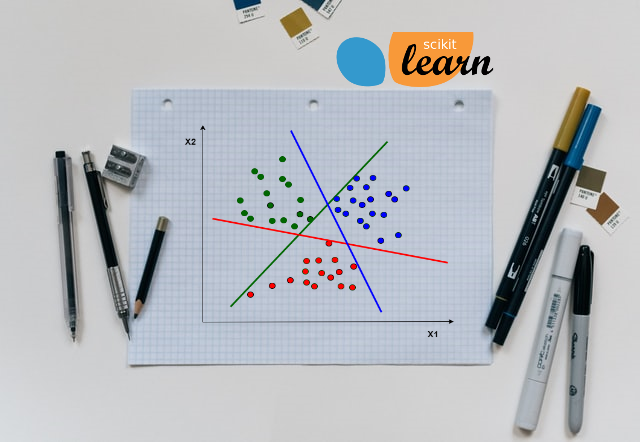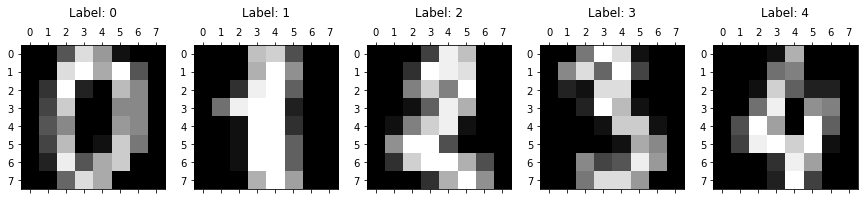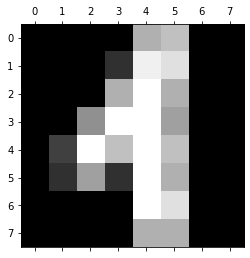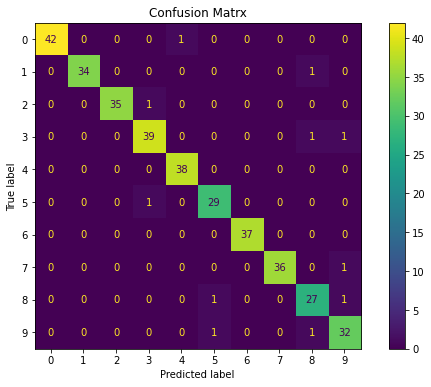# Multiclass Logistic Regression Using SklearnIn this study we are going to use the Linear Model from Sklearn library to perform Multi class Logistic Regression. We are going to use handwritten digit’s dataset from Sklearn. Optical recognition of handwritten digits dataset

# Introduction

When outcome has more than to categories, Multi class regression is used for classification. For e.g. mail classification as primary, social, promotions, forums.

We are going to use One Vs Rest (OVR) algorithm also known as one vs all algorithm. As name suggest in this algorithm we choose one class and put all other classes into second virtual class and run the binary logistic regression on it. We repeat this procedure for all the classes in the dataset. So we actually end up with binary classifiers designed to recognize each class in dataset

For prediction on given data, our algorithm returns probabilities for each class in the dataset and whichever class has the highest probability is our prediction

# Problem Statement

The data set contains images of hand-written digits: 10 classes where each class refers to a digit(0 to 9). Objective of our model is to predict the correct digit from 0 to 9, based on given handwritten image of digit.

# Import Libraries

• pandas: Used for data manipulation and analysis
• numpy : Numpy is the core library for scientific computing in Python. It is used for working with arrays and matrices.
• matplotlib : It’s plotting library, and we are going to use it for data visualization
• datasets: Here we are going to use ‘load_digits’ dataset
• model_selection: Here we are going to use model_selection.train_test_split() for splitting the data
• linear_model: Here we are going to linear_model.LogisticRegression() for classification
• metrics: Here we are going use metrics.plot_confusion_matrix() and metrics.classification_report() for model analysis
``````import pandas as pd
import numpy as np
import matplotlib.pyplot as plt
from sklearn import datasets
from sklearn import model_selection
from sklearn import linear_model
from sklearn import metrics
``````

# Load The Data

• We are going to use Optical recognition of handwritten digits dataset
• Dataset characteristics are,
• Number of Attributes: 64
• Attribute Information: 8x8 image of integer pixels in the range 0 to 16.
• Missing Attribute Values: None
• Creator: Alpaydin (alpaydin ‘@’ boun.edu.tr)
• Date: July; 1998
``````digits_df = datasets.load_digits()
print('Digits dataset structure= ', dir(digits_df))
print('Data shape= ', digits_df.data.shape)
print('Data conatins pixel representation of each image, \n', digits_df.data)
``````
``````Digits dataset structure=  ['DESCR', 'data', 'images', 'target', 'target_names']
Data shape=  (1797, 64)
Data conatins pixel representation of each image,
[[ 0.  0.  5. ...  0.  0.  0.]
[ 0.  0.  0. ... 10.  0.  0.]
[ 0.  0.  0. ... 16.  9.  0.]
...
[ 0.  0.  1. ...  6.  0.  0.]
[ 0.  0.  2. ... 12.  0.  0.]
[ 0.  0. 10. ... 12.  1.  0.]]
``````

# Data Understanding

Dataset contains 10 classes(0 to 9 digits). There are 180 training example per class and total 1797 training examples. Each training example is 8x8 image i.e. flat array of 64 pixels or matrix of 8x8. Each pixel value is represented by integer from 0 to 16. So our input data is of shape (1797x64) i.e. 1797 rows and 64 columns

• digits.DESCR: Description of the dataset
• digits.data:
• ndarray of shape (1797, 64)
• The flattened data matrix of training data.i.e Every 8x8 image data matrix is converted to 64 pixel flat array.
• We are going to use this data for model training
• digits.images:
• ndarray of shape (1797, 8, 8)
• It contains raw image data in the form of 8x8 matrix
• We are going to use this data for plotting the images
• digits.target: Contains target value(0 to 9) for each training examples, so it contains 1797, y labels
• digits.target_names: Contains name for each target since we have 10 classes it contains 10 names only

Here digits.data is our independent/inputs/ X variables

And digits.target is our dependent/target/y variable

## Data Visualization

Let’s visualize the images from digits dataset

``````# Using subplot to plot the digits from 0 to 4
rows = 1
columns = 5
fig, ax =  plt.subplots(rows, columns, figsize = (15,6))

plt.gray()
for i in range(columns):
ax[i].matshow(digits_df.images[i])
ax[i].set_title('Label: %s\n' % digits_df.target_names[i])

plt.show()
``````# Build Machine Learning Model

## Create Feature Matrix X and Label Vector y

Note for training and testing we are going to use ‘digits_df.data’ and not ‘digits_df.images’

``````X = digits_df.data
y = digits_df.target
``````

## Create Test And Train Dataset

• We will split the dataset, so that we can use one set of data for training the model and one set of data for testing the model
• We will keep 20% of data for testing and 80% of data for training the model
• If you want to learn more about it, please refer Train Test Split tutorial
``````X_train, X_test, y_train, y_test = model_selection.train_test_split(X, y, test_size= 0.2, random_state = 1)

print('X_train dimension= ', X_train.shape)
print('X_test dimension= ', X_test.shape)
print('y_train dimension= ', y_train.shape)
print('y_train dimension= ', y_test.shape)
``````
``````X_train dimension=  (1437, 64)
X_test dimension=  (360, 64)
y_train dimension=  (1437,)
y_train dimension=  (360,)
``````

Now lets train the model using OVR algorithm

## Multi class Logistic Regression Using OVR

• Since we are going to use One Vs Rest algorithm, set > multi_class=’ovr’
• Note: since we are using One Vs Rest algorithm we must use ‘liblinear’ solver with it.
``````lm = linear_model.LogisticRegression(multi_class='ovr', solver='liblinear')
lm.fit(X_train, y_train)
``````
``````LogisticRegression(C=1.0, class_weight=None, dual=False, fit_intercept=True,
intercept_scaling=1, l1_ratio=None, max_iter=100,
multi_class='ovr', n_jobs=None, penalty='l2',
random_state=None, solver='liblinear', tol=0.0001, verbose=0,
warm_start=False)
``````

# Testing The Model

• For testing we are going to use the test data only
• Question: Predict the value of 200 digits from test data
``````print('Predicted value is =', lm.predict([X_test]))

print('Actual value from test data is %s and corresponding image is as below' % (y_test) )
plt.matshow(digits_df.images)
plt.show()
``````
``````Predicted value is = 
Actual value from test data is 4 and corresponding image is as below
``````## Model Score

Check the model score using test data

``````lm.score(X_test, y_test)
``````
``````0.9694444444444444
``````

## Confusion Matrix

• Confusion matrix helps to visualize the performance of the model
• The diagonal elements represent the number of points for which the predicted label is equal to the true label
• Off-diagonal elements are those that are mislabeled by the classifier.
• The higher the diagonal values of the confusion matrix the better, indicating many correct

Let’s create confusion matrix using sklearn library and test data

``````#Creating matplotlib axes object to assign figuresize and figure title
fig, ax = plt.subplots(figsize=(10, 6))
ax.set_title('Confusion Matrx')

disp =metrics.plot_confusion_matrix(lm, X_test, y_test, display_labels= digits_df.target_names, ax = ax)
disp.confusion_matrix
``````
``````array([[42,  0,  0,  0,  1,  0,  0,  0,  0,  0],
[ 0, 34,  0,  0,  0,  0,  0,  0,  1,  0],
[ 0,  0, 35,  1,  0,  0,  0,  0,  0,  0],
[ 0,  0,  0, 39,  0,  0,  0,  0,  1,  1],
[ 0,  0,  0,  0, 38,  0,  0,  0,  0,  0],
[ 0,  0,  0,  1,  0, 29,  0,  0,  0,  0],
[ 0,  0,  0,  0,  0,  0, 37,  0,  0,  0],
[ 0,  0,  0,  0,  0,  0,  0, 36,  0,  1],
[ 0,  0,  0,  0,  0,  1,  0,  0, 27,  1],
[ 0,  0,  0,  0,  0,  1,  0,  0,  1, 32]])
``````## Classification Report

Classification report is used to measure the quality of prediction from classification algorithm

• Precision: Indicates how many classes are correctly classified
• Recall: Indicates what proportions of actual positives was identified correctly
• F-Score: It is the harmonic mean between precision & recall
• Support: It is the number of occurrence of the given class in our dataset
``````print(metrics.classification_report(y_test, lm.predict(X_test)))
``````
``````              precision    recall  f1-score   support

0       1.00      0.98      0.99        43
1       1.00      0.97      0.99        35
2       1.00      0.97      0.99        36
3       0.95      0.95      0.95        41
4       0.97      1.00      0.99        38
5       0.94      0.97      0.95        30
6       1.00      1.00      1.00        37
7       1.00      0.97      0.99        37
8       0.90      0.93      0.92        29
9       0.91      0.94      0.93        34

accuracy                           0.97       360
macro avg       0.97      0.97      0.97       360
weighted avg       0.97      0.97      0.97       360
``````

## Learning Path for DP-900 Microsoft Azure Data Fundamentals Certification

8 minute read

Learning path to gain necessary skills and to clear the Azure Data Fundamentals Certification. This certification is intended for candidates beginning to wor...

## Learning Path for AI-900 Microsoft Azure AI Fundamentals Certification

7 minute read

Learning path to gain necessary skills and to clear the Azure AI Fundamentals Certification. This certification is intended for candidates with both technica...

## ANN Model to Classify Images

12 minute read

In this guide we are going to create and train the neural network model to classify the clothing images. We will use TensorFlow deep learning framework along...

## Introduction to NLP

8 minute read

In short NLP is an AI technique used to do text analysis. Whenever we have lots of text data to analyze we can use NLP. Apart from text analysis, NLP also us...

## K Fold Cross Validation

14 minute read

There are multiple ways to split the data for model training and testing, in this article we are going to cover K Fold and Stratified K Fold cross validation...

## K-Means Clustering

13 minute read

K-Means clustering is most commonly used unsupervised learning algorithm to find groups in unlabeled data. Here K represents the number of groups or clusters...

## Time Series Analysis and Forecasting

10 minute read

Any data recorded with some fixed interval of time is called as time series data. This fixed interval can be hourly, daily, monthly or yearly. Objective of t...

## Support Vector Machines

9 minute read

Support vector machines is one of the most powerful ‘Black Box’ machine learning algorithm. It belongs to the family of supervised learning algorithm. Used t...

## Random Forest

12 minute read

Random forest is supervised learning algorithm and can be used to solve classification and regression problems. Unlike decision tree random forest fits multi...

## Decision Tree

13 minute read

Decision tree explained using classification and regression example. The objective of decision tree is to split the data in such a way that at the end we hav...

## Agile Scrum Framework

7 minute read

This tutorial covers basic Agile principles and use of Scrum framework in software development projects.

## Underfitting & Overfitting

2 minute read

Main objective of any machine learning model is to generalize the learning based on training data, so that it will be able to do predictions accurately on un...

## Multiclass Logistic Regression Using Sklearn

6 minute read

In this study we are going to use the Linear Model from Sklearn library to perform Multi class Logistic Regression. We are going to use handwritten digit’s d...

## Binary Logistic Regression Using Sklearn

5 minute read

In this tutorial we are going to use the Logistic Model from Sklearn library. We are also going to use the same test data used in Logistic Regression From Sc...

## Logistic Regression From Scratch With Python

13 minute read

This tutorial covers basic concepts of logistic regression. I will explain the process of creating a model right from hypothesis function to algorithm. We wi...

## Train Test Split

3 minute read

In this tutorial we are going to study about train, test data split. We will use sklearn library to do the data split.

## One Hot Encoding

11 minute read

In this tutorial we are going to study about One Hot Encoding. We will also use pandas and sklearn libraries to convert categorical data into numeric data.

## Multivariate Linear Regression Using Scikit Learn

8 minute read

In this tutorial we are going to use the Linear Models from Sklearn library. Scikit-learn is one of the most popular open source machine learning library for...

## Univariate Linear Regression Using Scikit Learn

7 minute read

In this tutorial we are going to use the Linear Models from Sklearn library. Scikit-learn is one of the most popular open source machine learning library for...

## Multivariate Linear Regression From Scratch With Python

10 minute read

In this tutorial we are going to cover linear regression with multiple input variables. We are going to use same model that we have created in Univariate Lin...

## Univariate Linear Regression From Scratch With Python

13 minute read

This tutorial covers basic concepts of linear regression. I will explain the process of creating a model right from hypothesis function to gradient descent a...

## Machine Learning Introduction And Learning Plan

4 minute read

In this tutorial we will see the brief introduction of Machine Learning and preferred learning plan for beginners

Back to top ↑